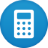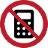# Vector subtraction

Description and examples for the subtraction of vectors

## Subtraction of vectors

The following article describes vector subtractions using vectors of two or three elements. Basically, vectors can contain any number of elements.

Vectors can be subtracted by adding the individual elements. However, vectors can only be subtracted if they have the same number of dimensions and the same orientation (columns or row-oriented).

The following vectors can be subtracted. They have the same number of elements and same orientation.$$\left[\matrix{X_a\\Y_a}\right] - \left[\matrix{X_b\\Y_b}\right]$$     and    $$\left[\matrix{X_a\\Y_a\\Z_a}\right] - \left[\matrix{X_b\\Y_b\\Z_b}\right]$$

The following vectors can not be subtracted because they have a different number of elements.$$\left[\matrix{X_a\\Y_a}\right] - \left[\matrix{X_b\\Y_b\\Z_b}\right]$$

The following vectors can not be subtracted because they have a different orientation.$$[X_a\;Y_a\;Z_a]- \left[\matrix{X_b\\Y_b\\Z_b}\right]$$

Examples

$$\left[\matrix{a\\b}\right] - \left[\matrix{c\\d}\right] = \left[\matrix{a-c\\b-d}\right]$$
$$\left[\matrix{3\\5}\right] - \left[\matrix{1\\4}\right] = \left[\matrix{3-1\\5-4}\right]=\left[\matrix{2\\1}\right]$$

The subtraction on vectors of higher dimension is according to the same principle.

$$\left[\matrix{a\\b\\c}\right] - \left[\matrix{x\\y\\z}\right] = \left[\matrix{a-x\\b-y\\c-z}\right]$$
$$\left[\matrix{10\\20\\30}\right] - \left[\matrix{1\\2\\3}\right] = \left[\matrix{10-1\\20-2\\30-3}\right] =\left[\matrix{9\\18\\27}\right]$$

## Graphic Vector Subtraction

The following figure shows the graphic vector subtraction of the expression

$$\left[\matrix{5\\5}\right] - \left[\matrix{4\\2}\right] = \left[\matrix{5-4\\5-2}\right]=\left[\matrix{1\\3}\right]$$

First the line of the first vector (red) is drawn from the zero point to the position $$x = 5, y = 5$$.

Then, from the top of the first vector, the second vector (yellow) is drawn to the position by 4 units to the left and 2 units to the bottom.

The sum vector (blue) is determined by the line from the base point of the first to the peak of the second vector

The addition of vectors is identical to the subtraction of vectors, but with positive operator. The same rules apply to the vector addition as to the vector subtraction.